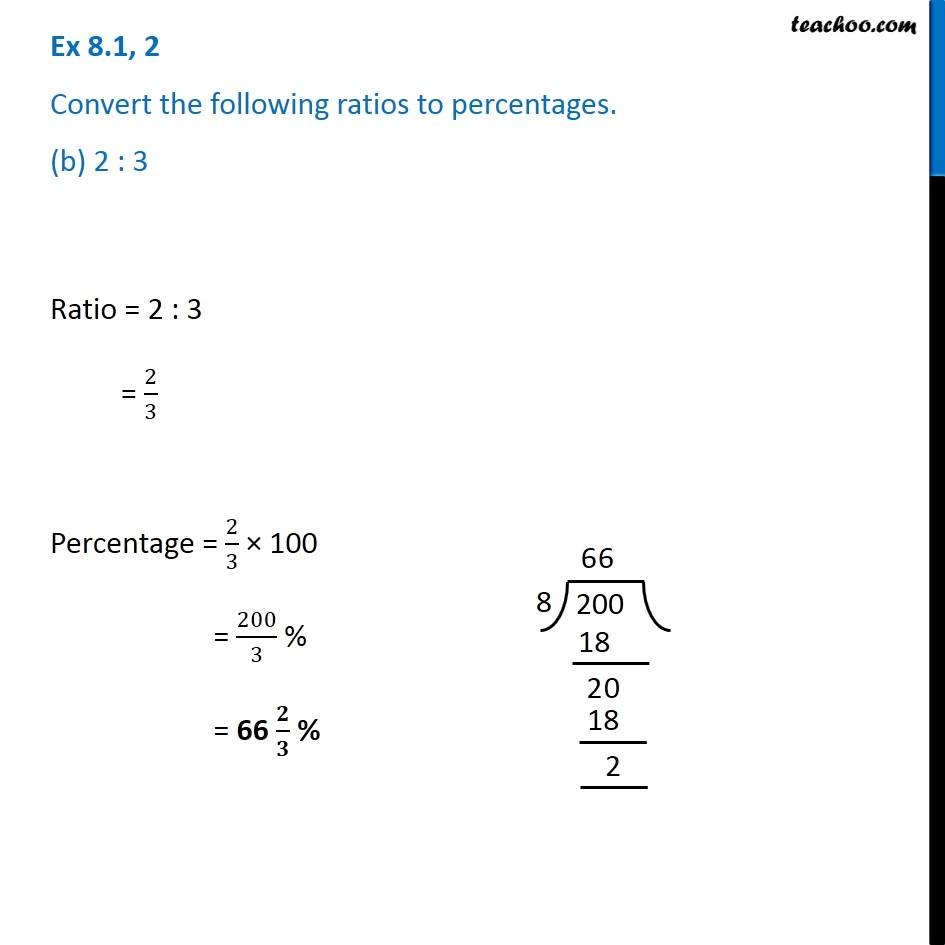Ex 8.1

Chapter 8 Class 8 Comparing Quantities
Serial order wiseGet live Maths 1-on-1 Classs - Class 6 to 12

### Transcript

Ex 8.1, 2 Convert the following ratios to percentages. (b) 2 : 3Ratio = 2 : 3 = 2/3 Percentage = 2/3 × 100 = 200/3 % = 66 𝟐/𝟑 %